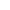Top | Introduction | Members | Activities | Call for Paper | Link |Japanese## "A study on Efficient Hyperelliptic Curve Exponentiation"

 Hyperelliptic curve cryptosystems are one of the most attractive next generation public-key cryptosystems. Exponetiation on hyperelliptic curve is the most important for hyperelliptic curve cryptosystems. In this paper, we apply the Mixed coordinates sysytem, which is an efficient exponentiation method on elliptic curve, to genus 2 hyperelliptic curve. We analyze the optimal coordinates of genus 2 hyperelliptic curves. [ back ]